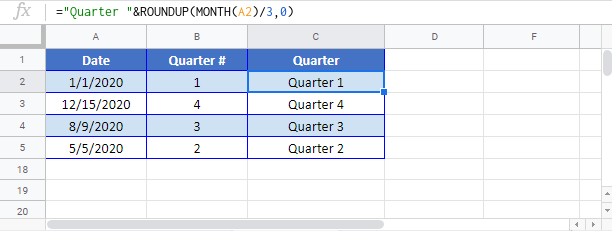### Calculate Quarter from a Date – Excel & Google Sheets

This tutorial will demonstrate how to calculate the quarter from a date.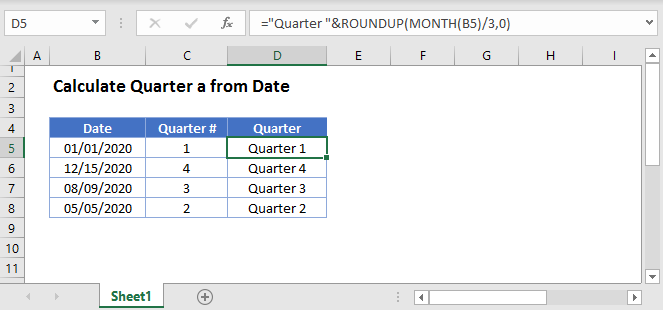## Quarter of a Date

To calculate the quarter from a date, first we want to get the month number: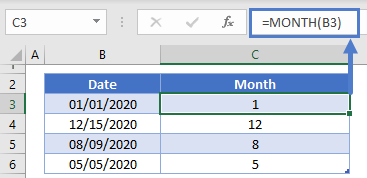Next we want to divide the month number by 3 (each quarter has 3 months) and use the ROUNDUP Function to round the result up to the nearest whole integer: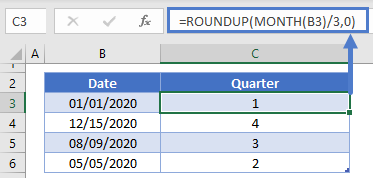Next, we can concatenate text to the front of the result to say “Q1”, “Q2”, etc.: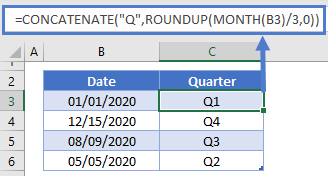or “Quarter 1”, “Quarter 2”, etc.

## Calculate Quarter from a Date in Google Sheets

All of the above examples work exactly the same in Google Sheets as in Excel.